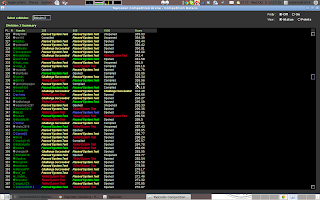## Wednesday, October 5, 2011

### SRM520

Level 1 Level 2 Level 3
Div 1 Level 1 Level 2 Level 3
Div 2 Level 1 Level 2 Level 3SRM520
My rank: 370 - 280 - 368.
which takes me back to green.
I think this contest is really a good practice for dynamic programming, for both div1 and div2.

## Tutorials:

### Solution

The same with Div2 - Level 2.

### Solution

Dynamic programming, at the pos-th candidate, as long as the passed number is greater than the pos+1 th candidate, it will be find, or if the have tie for the number of passed, then could compare the number of challenged. aa, bb, cc are the choice we have for each problem, for each choice we could check the next candidate.
dp[pos] [pass][cha] represents the way that pos-th candidate have pass number of passed problems, while cha number of challenged problems.
The comments I put there before three nested for loops, is the alternative way I though works, but it seems not work well, I could not figure it out why. Hope somebody could help me with this, or I could figure it out later.

### Source Code:

//Tue Oct  4 14:45:42 PDT 2011
public class SRMSystemTestPhase {

public String[] record;
public int[][][] dp;
public static final int mod = 1000000007;

public int countWays(String[] description) {
record = description;
dp = new int[description.length + 1];

for (int i = 0; i <= description.length; i++)
for (int j = 0; j < 4; j++)
for (int k = 0; k < 4; k++)
dp[i][j][k] = -1;

return solve(0, 3, 0);
}

public int solve(int pos, int pass, int cha) {
if (pos == record.length)
return 1;
if (dp[pos][pass][cha] > -1)
return dp[pos][pass][cha];
int ret = 0;
int aa = record[pos].charAt(0) == 'Y' ? 3 : 1;
int bb = record[pos].charAt(1) == 'Y' ? 3 : 1;
int cc = record[pos].charAt(2) == 'Y' ? 3 : 1;

// 0:pass;
// 2:failed;

// int passed = 0;
// for (int i = 0; i < 3; i++) {
// if (record[pos].charAt(i) == 'Y')
// if ((1 & (mask >> i)) == 1) {
// passed++;
// } else
// }
// if (passed < pass || (passed == pass && chad >= cha))
// ret = (ret + solve(pos + 1, passed, chad)) % mod;
// }
for (int a = 0; a < aa; a++)
for (int b = 0; b < bb; b++)
for (int c = 0; c < cc; c++) {
int passed = 0;
if (record[pos].charAt(0) == 'Y' && a == 0)
passed++;
if (record[pos].charAt(0) == 'Y' && a == 1)
if (record[pos].charAt(1) == 'Y' && b == 0)
passed++;
if (record[pos].charAt(1) == 'Y' && b == 1)
if (record[pos].charAt(2) == 'Y' && c == 0)
passed++;
if (record[pos].charAt(2) == 'Y' && c == 1)
if (passed < pass || (passed == pass && chad >= cha))
ret = (ret + solve(pos + 1, passed, chad)) % mod;
}
return dp[pos][pass][cha] = ret;
}
// <%:testing-code%>
}

### Solution

Brute-force all of the solutions, then check. Logic problems bothers me a lot for this problem.

### Source Code:

//Tue Oct  4 14:43:37 PDT 2011
import java.util.*;
import java.util.regex.*;
import java.text.*;
import java.math.*;
import java.awt.geom.*;

public class SRMCodingPhase {
public int countScore(int[] points, int[] skills, int lluck) {
int pts = 0;
for (int i = 1; i < (1 << 3); i++) {
int tmp = 0;
int luck = lluck;
int min = 75;

for (int j = 2; j >= 0; j--) {
if ((i & (1 << j)) > 0) {
if ((luck == 0 && min < skills[j])
|| (luck != 0 && min < (luck >= skills[j] ? 1
: skills[j] - luck)))
break;

tmp += points[j] - (1 << (j + 1)) * skills[j];
min -= skills[j];
if (luck > 0) {
if (luck < skills[j]) {
tmp += (1 << (j + 1)) * luck;
min += luck;
luck = 0;

} else {
tmp += (1 << (j + 1)) * (skills[j] - 1);
luck -= skills[j] - 1;
min += skills[j] - 1;
}
}
// if(i==3)
// System.out.println(min);
}
}
pts = Math.max(pts, tmp);
}
return pts;
}
}

### Source Code:

//Tue 04 Oct 2011 14:30:27 PDT
import java.util.*;
import java.util.regex.*;
import java.text.*;
import java.math.*;
import java.awt.geom.*;

public class SRMRoomAssignmentPhase {
public int countCompetitors(int[] ratings, int K) {
int count = 0;
for (int r : ratings) {
if (r > ratings)
count++;
}
return count / K;
}
}